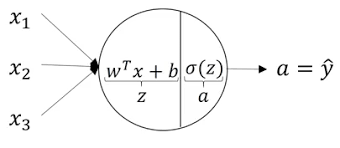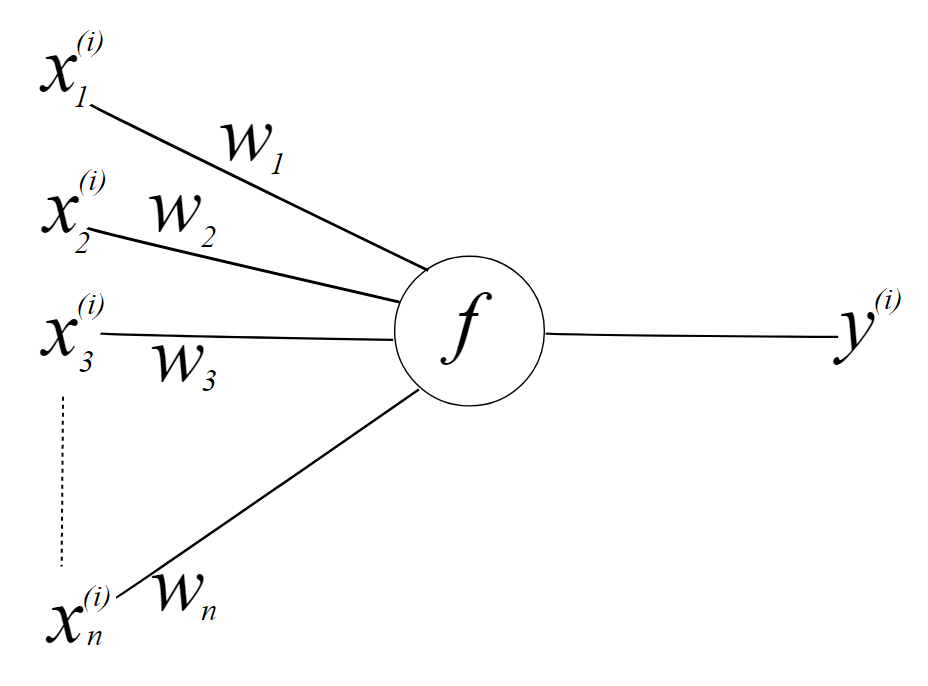# Implementing logistic regression as a neural network from scratch

I recently started exploring the world of Deep Learning and this process of converting a classical Machine Learning problem (Logistic Regression) into a Deep Learning problem (Logistic Regression using neural networks) was just so satisfying that I had to write a blog about it, so here it goes. Below are a couple of visual representations of the neural network that we will be creating -Neural network with a single neuron — stuff happening inside a neuron (reference)Neural network with a single neuron — features, weights, and the final output (reference)

We will be coding out a python class from scratch to implement the algorithm explained below.

# Understanding Mathematics a bit

I hope that you do understand the basics of a neural network, so I’ll go through the mathematics required for this blog quickly. Most of the images below have been derived from Andrew Ng’s Deep Learning course -

## The logistic regression algorithm

We start by writing down the algorithm's mathematics and defining a linear function with random weights (equal to the number of features provided) and a bias to fit onto our data. This can be thought of as fitting a straight line on our data if the number of features (x) is one.

We then pass the output of this linear function into a non-linear function (here a sigmoid function) to squish the output between 0 and 1 (kind of like probability).

The next thing we need is a loss function to calculate how well our algorithm is doing, and we choose the standard one (binary cross-entropy loss or log loss) which is then summed up over the data to calculate the cost function.

The last thing we need here is backpropagation, where we calculate the partial derivates (for gradient descent) in the opposite direction of the conventional flow. I’ll suggest 3Blue1Brown’s video for this particular topic.

Our next step would be to fit our data by running the classic gradient descent algorithm. For this, we write a pseudocode for the backpropagation step shown above to compute all the required derivatives. Then we use these derivatives and the learning rate to adjust our parameters over several epochs (or till our cost function converges).

## Vectorizing logistic regression

Our final step would be to make our algorithm efficient by getting rid of the for loops. We do so by treating everything as matrices so that we can perform matrix operations on the whole dataset in a single go using `numpy` .

# The code

Finally, we’ll implement the algorithm explained above step by step in python.

## Sigmoid function

Starting with the easiest bit, let us create the sigmoid function (a class method).

## Building the class

Next, let us build a class that accepts features, labels, and some other required items.

## Fitting the data

Now comes the main part of the code, the `fit` method. We start by initializing the cost function (J) and the previously seen cost function (J_last).

Next, we create an empty array for `W` (weights), `dW` (a derivative of the loss function w.r.t. weights) and create an infinite loop that breaks when the cost function starts converging (when the difference in 2 consecutive values of the cost function is less than `1e-5`).

The loop performs all the mathematics explained above and finds the appropriate weights for our data (by running gradient descent).

## Predicting

Finally, now we can create a `predict` method to utilize the calculated weights for predicting outputs. For this, we take in a list of list of features and iterate through each data to assign it either 1 or 0 using our good old sigmoid function.

## The complete code

The complete class with some predictions -

Hope you had fun with all the mathematics and python:)